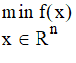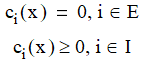# NMath User's Guide

30.2 Nonlinear Programming (.NET, C#, CSharp, VB, Visual Basic, F#)

A general formulation of a nonlinear programming (NLP) problem is:subject towhere the functions f and ci are all smooth (continuous derivative), real-valued functions on a subset of Rn, and E and I are finite sets of indices. Function f is called the objective function, and functions ci are called the constraint functions.

Encapsulating the Problem

In NMath, class encapsulates an NLP problem. encapsulates an NLP which may contain integral or binary constraints.

Instances are constructed from an objective function to minimize, and optionally an IEnumerable of Constraint objects. Alternatively, constraints can be added post-construction using convenience methods.

For example, if MyObjectiveFunction extends DoubleFunctional (see Section 30.1):

Code Example – C# nonlinear programming

```DoubleFunctional objective = new MyObjectiveFunction();
```
```var problem = new NonlinearProgrammingProblem( objective );
```

Code Example – VB nonlinear programming

```Dim Objective As DoubleFunctional = New MyObjectiveFunction()
```
```Dim Problem As New NonlinearProgrammingProblem(Objective)
```

Rather than sub-classing, you can also use a delegate to express the objective function, in which case you must also specify the dimension of the domain of the objective function. For instance:

Code Example – C# nonlinear programming

```public double MyFunction( DoubleVector x )
```
```{
```
```  // min f(x) = -x0 * x1 * x2
```
```  return -x * x * x;
```
```}

```

```int xDim = 3;
```
```Func<DoubleVector, double> objective = MyFunction;
```
```var problem = new NonlinearProgrammingProblem( xDim, objective );
```

Code Example – VB nonlinear programming

```Public Function MyFunction(X As DoubleVector)
```
```  ' min f(x) = -x0 * x1 * x2
```
```  Return -X(0) * X(1) * X(2)
```
```End Function

```

```Dim XDim As Integer = 3
```
```Dim Objective As New Func(Of DoubleVector, Double)(MyFunction)
```
```Dim Problem As New NonlinearProgrammingProblem(XDim, Objective)
```

This code specifies two constraints in the constructor:

Code Example – C# nonlinear programming

```var constraints = new List<Constraint>();
```
```var c1 =
```
```    new DoubleFunctionalDelegate( 2, new Func<DoubleVector,
```
```        double>(delegate(DoubleVector v) { return v; }) );
```
```var constraint1 = new NonlinearConstraint(
```
```    c1, ConstraintType.GreaterThanOrEqualTo )
```
```constraints.Add( constraint1 );

```

```var c2 =
```
```    new DoubleFunctionalDelegate(2, new Func<DoubleVector,
```
```        double>(delegate(DoubleVector v) { return v; }));
```
```var constraint2 = new NonlinearConstraint(
```
```    c2, ConstraintType.GreaterThanOrEqualTo )
```
```constraints.Add( constraint2 );

```

```var problem =
```
```    new NonlinearProgrammingProblem( objective, constraints );
```

Code Example – VB nonlinear programming

```Dim Constraints As New List(Of Constraint)
```
```Dim C1 As New DoubleFunctionalDelegate(2,
```
```  New Func(Of DoubleVector, Double)(MyConstraintFunction1))
```
```Dim Constraint1 As New NonlinearConstraint(C1,
```
```  ConstraintType.GreaterThanOrEqualTo)
```
```Constraints.Add(Constraint1)

```

```Dim C2 As New DoubleFunctionalDelegate(2,
```
```  New Func(Of DoubleVector, Double)(MyConstraintFunction2))
```
```Dim Constraint2 As New NonlinearConstraint(C2,
```
```  ConstraintType.GreaterThanOrEqualTo)
```
```Constraints.Add(Constraint2)

```

```Dim Problem As New NonlinearProgrammingProblem(Objective,
```
```  Constraints)
```

Class NonlinearProgrammingProblem provides several convenience methods for adding constraints and variable bound to an existing problem object.

For example, this code adds lower and upper variable bounds:

Code Example – C# nonlinear programming

```// 0 <= x0, x1, x2 <= 42
```
```for ( int i = 0; i < xDim; i++ ) {
```
```    problem.AddBounds( i, 0.0, 42.0 );
```
```}
```

Code Example – VB nonlinear programming

```' 0 &lt= x0, x1, x2 &lt= 42
```
```For I As Integer = 0 To XDim - 1
```
```  Problem.AddBounds(I, 0.0, 42.0)
```
```Next
```

This code adds a linear constraint:

Code Example – C# nonlinear programming

```// 0 <= x0 + 2*x1 + 2*x2 <= 72,
```
```problem.AddLinearConstraint( new DoubleVector( 1.0, 2.0, 2.0 ),
```
```    0.0, 72 );
```

Code Example – VB nonlinear programming

```' 0 &lt= x0 + 2*x1 + 2*x2 &lt= 72,
```
```Problem.AddLinearConstraint(New DoubleVector(1.0, 2.0, 2.0),0.0,
```
```  72)
```

Code Example – C# nonlinear programming

```int xDim = 2;

```

```// x0*x1 >= -10
```
```problem.AddLowerBoundConstraint( xDim,
```
```    ( DoubleVector x ) => x * x, -10.0 );

```

```// x0*x1 - x0 -x1 <= -1.5
```
```problem.AddUpperBoundConstraint( xDim,
```
```    ( DoubleVector x ) => x * x - x - x, -1.5 );
```

Code Example – VB nonlinear programming

```Dim XDim As Integer = 2

```

``` Public Function LowerConstraint(X As DoubleVector) As Double
```
```  Return X(0) * X(1)
```
```End Function

```

```Public Function UpperConstraint(X As DoubleVector) As Double
```
```  Return X(0) * X(1) - X(0) - X(1)
```
```End Function
```
```
```
```' x0*x1 &gt= -10
```
```Problem.AddLowerBoundConstraint(xDim, New Func(Of DoubleVector,
```
```  Double)(AddressOf LowerConstraint), -10.0)

```

```' x0*x1 - x0 -x1 &lt= -1.5
```
```Problem.AddUpperBoundConstraint(xDim, New Func(Of DoubleVector,
```
```  Double)(AddressOf UpperConstraint), -1.5)
```

encapsulates an NLP which may contain integral or binary constraints. For example, in this code variable index 2 is constrained to be integer valued.

Code Example – C# integer programming

```problem.AddIntegralConstraint( 2 );
```

Here, variable indices 0 and 1 must be binary.

Code Example – C# binary programming

```problem.AddBinaryConstraint( 0, 1 );
```

A binary constraint restricts the variable to a value of zero or one.

Method GetIntegrality() gets the integral constraint state of the variable at the given index. IntegralVariableIndices returns the indices of variables with integral constraints.

Solving the Problem

NMath provides two types of NLP solvers: Stochastic Hill Climbing and Sequential Quadratic Programming (SQP).

Stochastic Hill Climbing

The strategy of the Stochastic Hill Climbing algorithm is to iteratively make small random changes to the decision values. A candidate solution is accepted if it results in an improvement, and rejected if it makes it worse. The strategy addresses the limitations of deterministic hill climbing techniques, which are prone to getting stuck in local optima due to their greedy acceptance of neighboring moves.

solves NLP problems using the Stochastic Hill Climbing algorithm.

Code Example – C# nonlinear programming

var solver = new StochasticHillClimbingSolver();

Code Example – VB nonlinear programming

```Dim Solver As New StochasticHillClimbingSolver()
```

The algorithm is stochastic. Setting a random seed ensures consistent results between runs.

Code Example – C# nonlinear programming

```solver.RandomSeed = 0x248;
```

Code Example – VB nonlinear programming

```Solver.RandomSeed = &H248
```

Additional parameters are specified using an instance of StochasticHillClimbingParameters.

Code Example – C# nonlinear programming

```var solverParams = new StochasticHillClimbingParameters
```
```{
```
```  TimeLimitMilliSeconds = 10000,
```
```  Presolve = true
```
```};
```

Code Example – VB nonlinear programming

```Dim SolverParams As New StochasticHillClimbingParameters()
```
```SolverParams.TimeLimitMilliSeconds = 10000
```
```SolverParams.Presolve = True
```

Note that this example sets a time limit of 10 seconds for the solver. By default, the solver runs until a solution is found. Since this may take forever, it is a good idea to set a reasonable time limit on the solve. If an optimal solution is not found within the specified time limit, the solver exits and the solver's Result property will be equal to SolverResult.SolverInterrupted.

This example also sets Presolve = true. By default there is no pre-solve step. For some problems pre-solve can reduce the size and complexity and result in fewer steps to reach a solution.

This code performs the actual solve and prints out the results:

Code Example – C# nonlinear programming

solver.Solve( problem, solverParams );

```Console.WriteLine( "Solver Result = " + solver.Result );
```
```Console.WriteLine( "Number of steps = " + solver.Steps );
```
```Console.WriteLine( "Optimal x = " + solver.OptimalX );
```
```Console.WriteLine( "Optimal function value = " +
```
```  solver.OptimalObjectiveFunctionValue );
```

Code Example – VB nonlinear programming

```Solver.Solve(Problem, SolverParams)
```
```Console.WriteLine("Solver Result = {0}", Solver.Result)
```
```Console.WriteLine("Number of steps = {0}", Solver.Steps)
```
```Console.WriteLine("Optimal x = {0}", Solver.OptimalX)
```
```Console.WriteLine("Optimal function value = {0}",
```
```  Solver.OptimalObjectiveFunctionValue)
```

SQP algorithms solve NLP problems iteratively. At each step, a quadratic sub-problem is formed from the Hessian of the Lagrangian, Hk, the constraints, and the current iterate value xk. The solution of this sub-problem yields a step direction pk. Next a step size ak is determined, and the new iterate value is obtained as xk+1=xk+ak pk.

is the abstract base class for SQP solvers. NMath currently provides one concrete implementation: solves NLP problems using an active set algorithm.

Code Example – C# nonlinear programming

```var solver = new ActiveSetLineSearchSQP();
```

Code Example – VB nonlinear programming

```Dim Solver As New ActiveSetLineSearchSQP()
```

A convergence tolerance and maximum number of iterations can also be specified in the constructor, as well as other advanced options (see below):

Code Example – C# nonlinear programming

```double tolerance = 1e-4;
```
```var solver = new ActiveSetLineSearchSQP( tolerance );
```

Code Example – VB nonlinear programming

```Dim Tolerance As Double = "1e-4"
```
```Dim Solver As New ActiveSetLineSearchSQP(Tolerance)
```

The Solve() method solves the problem given an initial starting position, and returns true if the algorithm terminated successfully:

Code Example – C# nonlinear programming

```var x0 = new DoubleVector( 3, 1.0 );
```
```bool success = solver.Solve( problem, x0 );
```
```Console.WriteLine( "Termination status = " +
```
```    solver.SolverTerminationStatus );
```

Code Example – VB nonlinear programming

```Dim X0 As New DoubleVector(3, 1.0)
```
```Dim Success = Solver.Solve(Problem, X0)
```
```Console.WriteLine("Termination status = {0}",
```
```  Solver.SolverTerminationStatus)
```

Properties on the solver get the minimum x-value found, and the objective function evaluated at that point:

Code Example – C# nonlinear programming

```Console.WriteLine( "X = " + solver.OptimalX );
```
```Console.WriteLine( "f(x) = " +
```
```  solver.OptimalObjectiveFunctionValue );
```

Code Example – VB nonlinear programming

```Console.WriteLine("X = {0}", Solver.OptimalX)
```
```Console.WriteLine("f(x) = {0}",
```
```   Solver.OptimalObjectiveFunctionValue)
```

ActiveSetLineSearchSQP.Options provides advanced options for controlling the ActiveSetLineSearchSQP algorithm, such as the step size and finer grain convergence tolerances:

Code Example – C# nonlinear programming

```var solverOptions = new ActiveSetLineSearchSQP.Options();
```
```solverOptions.StepSizeCalculator = new ConstantSQPStepSize( 1 );
```
```solverOptions.StepDirectionTolerance = 1e-8;
```
```solverOptions.FunctionChangeTolerance = 1e-6;

```

```var solver = new ActiveSetLineSearchSQP( solverOptions );
```

Code Example – VB nonlinear programming

```Dim SolverOptions As New ActiveSetLineSearchSQP.Options()

```

```SolverOptions.StepSizeCalculator = New ConstantSQPStepSize(1)
```
```SolverOptions.StepDirectionTolerance = "1e-8"
```
```SolverOptions.FunctionChangeTolerance = "1e-6"

```

```Dim Solver As New ActiveSetLineSearchSQP(SolverOptions)
```

This code sets the step size calculator to use an instance of ConstantSQPStepSize, which simply returns a constant step size regardless of iteration values. By default, the ActiveSetLineSearchSQP algorithm uses an instance of L1MeritStepSize, which computes the step size based on sufficient decrease in the L1 merit function.

Top

Top